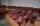Trucks

Three lorries droved bricks. One drove n bricks at once, second m less bricks than the first and third 300 bricks more the first lorry. The first lorry went 4 times a day the largest went 3 times a day and the smallest 5 times a day.

How many bricks brought:

a / all lorries in one day.
b / all lorries per week / Sunday don't worked /?

Result

a = (Correct answer is: 12n-5m+900)b = (Correct answer is: 72n-30m+5400)Solution:Leave us a comment of this math problem and its solution (i.e. if it is still somewhat unclear...):Be the first to comment!Next similar examples:

1. Theorem proveWe want to prove the sentence: If the natural number n is divisible by six, then n is divisible by three. From what assumption we started?
2. Holidays - on poolChildren's tickets to the swimming pool stands x € for an adult is € 2 more expensive. There was m children in the swimming pool and adults three times less. How many euros make treasurer for pool entry?
3. CandiesIn the box are 12 candies that look the same. Three of them are filled with nougat, five by nuts, four by cream. At least how many candies must Ivan choose to satisfy itself that the selection of two with the same filling? ?
4. TrigonometryIs true equality? ?
5. AlgebraX+y=5, find xy (find the product of x and y if x+y = 5)
6. Expressions 3If k(x+6)= 4x2 + 20, what is k(10)=?
7. Functions f,gFind g(1) if g(x) = 3x - x2 Find f(5) if f(x) = x + 1/2
8. Expression with powersIf x-1/x=5, find the value of x4+1/x4
9. CoefficientDetermine the coefficient of this sequence: 7.2; 2.4; 0.8
10. Quadratic function 2Which of the points belong function f:y= 2x2- 3x + 1 : A(-2, 15) B (3,10) C (1,4)
11. TeamsHow many ways can divide 16 players into two teams of 8 member?
12. SequenceWrite the first 6 members of these sequence: a1 = 5 a2 = 7 an+2 = an+1 +2 an
13. Elimination methodSolve system of linear equations by elimination method: 5/2x + 3/5y= 4/15 1/2x + 2/5y= 2/15
14. Third memberDetermine the third member of the AP if a4=93, d=7.5.
15. SequenceWrite the first 7 members of an arithmetic sequence: a1=-3, d=6.
16. AsymptoteWhat is the vertical asymptote of ?
17. ChairsDetermine the number of seats in the seventh row and ninth row, if 3rd row has 14 seats and in every next row of seats has five more than the previous row.